• 来源：机器学习算法与自然语言处理吴恩达新书《Machine Learning Yearning》完整中文版开源，整理给大家。书籍特色《Machine Learning Y...

来源：机器学习算法与自然语言处理

吴恩达新书《Machine Learning Yearning》完整中文版开源，整理给大家。

书籍特色

《Machine Learning Yearning》是吴恩达历时两年，根据自己多年实践经验整理出来的一本机器学习、深度学习实践经验宝典。作为一本 AI 实战圣经，本书主要教你如何在实践中使机器学习算法的实战经验。书籍PDF获取

在下方的公众号后台回复：MLY?关注“Python与人工智能社区”

展开全文• 最近在学习吴恩达机器学习课程，觉得讲得有理有据、循序渐进，很多问题豁然开朗，实践是检验真理的唯一标准，为了检验自己的所学所得，也为了更深一步地理解其原理，决定找个数据集来一波实战操作，记录于此一是...
最近在学习吴恩达的机器学习课程，觉得讲得有理有据、循序渐进，很多问题豁然开朗，实践是检验真理的唯一标准，为了检验自己的所学所得，也为了更深一步地理解其原理，决定找个数据集来一波实战操作，记录于此一是为了和广大机器学习的学者们分享，其次主要还是记录自己的学习过程，以稳步前行。首次涉及数据实战，代码和思路之中有许多不足之处，还请看官指正。
一、线性回归
线性回归是吴恩达老师的机器学习课程中的第一个入门算法，算法原理简单，容易理解。假设某数据共有m个特征和1个待预测特征y，为了能够通过推测特征y的值，假设与y的函数关系如下：

函数为线性函数，且具有多个变量，顾称之为多元线性回归，为找到能够尽量贴切地描述与y之间关系的函数，只需确定参数，为了描述预测值与实际值y之间的差异，引进代价函数如下：

是关于参数的函数，为使最大程度地接近y，即求使代价函数最小化的变量。
而中参数则由梯度下降算法决定，梯度下降算法通过不断地迭代更新值，使趋于最小化，更新规则为：

其中为学习率，若学习率过大可能会使梯度下降算法无法正常工作，若学习率过小则会使梯度下降过慢，增加迭代次数。当随着迭代次数增加，的下降趋于平缓时，梯度下降算法完成，此时的即为所求。
补充：为了使正常地更新，在中添加一个恒为1的特征，即。

二、了解数据
在uci网站上找到一个符合要求的数据集Abalone Data Set，数据集链接http://archive.ics.uci.edu/ml/datasets/Abalone。其属性信息如下：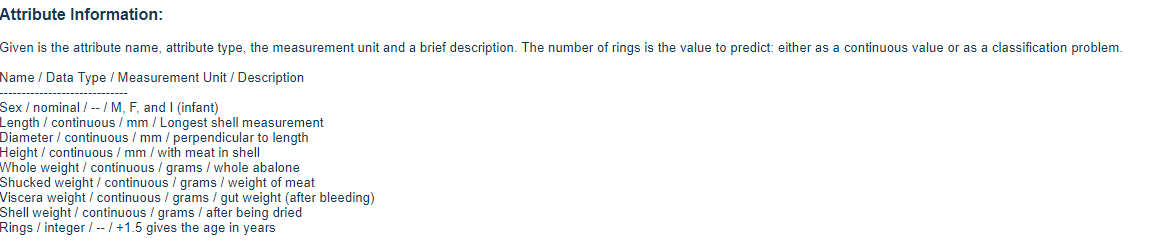数据集中共包含9个特征，分别是{'性别','壳体最长长度','直径','高度','全重','去壳重量','内脏重量','壳体重量','环数'}，其中性别(Sex)为分类特征，共有M\F\I三种属性，环数(Rings)是我们需要预测的特征，其余特征均为数值属性，我们需要做的工作就是根据样本的前8个特征，猜测其Rings属性值，Let's do it！

三、数据预处理
在编写算法之前，先需要对数据进行检查，看数据中有无缺失、是否符合我们所需的规范，实际上这部分的工作是及其麻烦的，好在本数据集已经为我们删除了有缺失值得样本，甚至已经将数据归一化处理，但为了确保算法正常运行，仍需检查数据。
1.读取数据
首先读取数据，查看数据维度，输出数据集前几个样本看是否读取成功。
#读取数据
import numpy as np

print(abalone_data.shape)
print(abalone_data[:10])
输出如下，可以看出数据读取正常：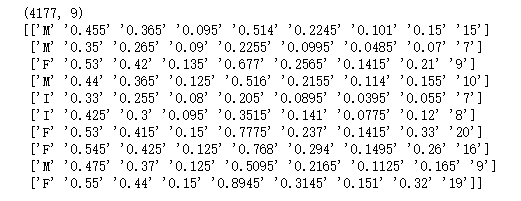2.检查数据
根据数据集的描述，我们需检查一下三点：
①性别属性是否只包含M、F、I三种
②数据项中是否具有非法字符，即数据中是否有代表缺失值的符号、是否是合法的浮点数
③根据属性的物理意义，显然这些属性的值都应为大于零的数。
#检查数据
#数据含义：{'性别','壳体最长长度','直径','高度','全重','去壳重量','内脏重量','壳体重量','环数'}

#1.检查性别是否只包含'M','F','I'三种类型
print('Sex Type:',set(abalone_data[:,0]))

#2.检查其余数值项是否包含非法字符
error_number = 0
for rows in abalone_data[:,1:]:
for sample in rows:
try:
float(sample)
except:
error_number += 1
print(rows)
print('Error Number:',error_number)

#3.检查数值项是否符合实际意义
abalone_data_number = abalone_data[:,1:].astype(np.float)
for rows in abalone_data_number>=0.0:
if False in rows:
print('rows')
上述代码运行结果如下：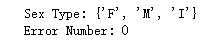可以确定，①Sex属性中只包括F、M、I三种值，②数据中不存在非法字符，③数据均符合其物理含义。检查数据完毕，接下来就可以使用它们了。
3.整理数据
注意到数据中sex为分类特征，在此将其简单处理，映射成整数项，即M-1，F-2，I-3。此外在上面提到的，为了使正常更新，在x中添加一个恒为1的属性列，最后，将整个数据集按4:1的比例划分为训练集和测试集，当然也可以用其他的方法。
经过运行下面的代码，我们得到了变量x的训练集X_train，预测值y的训练集Y_train，变量x的测试集X_test，预测值y的测试集Y_test。
#整理数据
#1.将sex属性转化为数值项   1-M  2-F  3-I
abalone_sex_col = abalone_data[:,0]
d = {'M':1,'F':2,'I':3}
abalone_sex_col = np.array([[d.get(x)] for x in abalone_sex_col])

#2.获取X
X = np.hstack((abalone_sex_col,abalone_data_number[:,:-1]))
x_0 = [] * X.shape
X = np.hstack((x_0,X))   #为每个样本添加一个恒为1的属性x_0

#3.获取Y
Y = abalone_data_number[:,-1]

#4.获取X和Y训练集测试集
sample_num = int(len(X) * 0.8)
X_train = X[:sample_num]
Y_train = Y[:sample_num]
X_test = X[sample_num:]
Y_test = Y[sample_num:]
四、算法实现
1.训练模型
接下来就简单了，根据原理里面介绍的，首先构建假设函数( 代码中为H(theta,X_row) )，然后构建代价函数（代码中为J（theta）），最后构建每次迭代时的更新函数（代码中为theta_update（alpha,theta_old））。
那么初始的和学习率该如何设置呢，由于梯度下降会不断更新值，且也会被人工调整，那就随机都设置成1好了，首先我们需要确定合适的学习率，在代码中可以这么设置：先运行较小的迭代次数如50次，观察代价函数是否随着迭代过程逐渐减小，选取能使持续减小的最大值，确定值后，适当增加迭代次数，以使梯度下降到最小值。
下面代码中的和迭代次数是多次调整后所得，在代码中绘制了随迭代次数增加的变化过程。此外还将迭代完成后的值输出，以查看函数和数据的拟合情况。
import matplotlib.pyplot as plt
#1.建立假设模型
###输入：theta向量，一个X样本
###输出：预测值
def H(theta,X_row):
mul = map(lambda x,y:x*y, theta, X_row)
return sum(mul)

#2.建立代价函数
###输入：theta向量
###输出：代价值
def J(theta):
dis = 0
for X_row,y in zip(X_train,Y_train):
dis += (H(theta,X_row)-y)**2
return dis/(2*len(X_train))

#3.theta向量更新函数
###输入：学习率alpha,待更新theta向量
###输出：更新后theta向量
def theta_update(alpha,theta_old):
theta_new = theta_old.copy()
for i in range(len(theta_new)):
temp = 0
for X_row,y in zip(X_train,Y_train):
temp += (H(theta_old,X_row) - y) * X_row[i]
temp /= len(X_train)
theta_new[i] = theta_old[i] - (alpha*temp)
return theta_new

#设置初始theta向量
theta_init =  * X_train.shape
alpha_init = 0.3
J_list = [J(theta_init)]
for i in range(1500):
theta_new = theta_update(alpha_init,theta_init)
J_list.append(J(theta_new))
theta_init = theta_new
print(J_list[-1])
plt.plot(J_list)
plt.show()
上述代码运行结果如下：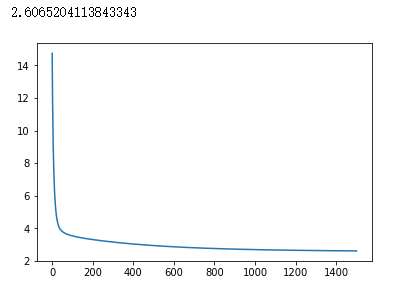可以看到在设置学习率为0.3，迭代1500次后得到的使整个训练集的代价函数减小到2.6065，到此，我们可以认为模型训练结束，即可根据此线性模型进行测试了，
2.测试
应用上一步训练得到的模型对测试集进行测试，代码如下：
forecase = []
for x in X_test:
forecase.append(round(H(theta_init,x)))  #获取测试数据预测值，由于预测的环数为整数，故进行四舍五入处理
confidence_interval = 2  #允许的误差范围
D_value_arr = np.array(forecase) - np.array(Y_test)  #获取预测值与真实值的差值数组
right_num = 0   #正确的个数（在允许误差范围内的个数）
for D_val in D_value_arr:
if D_val>= -confidence_interval and D_val <= confidence_interval:   #预测值在允许误差范围内
right_num += 1
print(right_num/(len(forecase)))

plt.figure(figsize=(20, 10))
coor_x = [i for i in range(len(forecase))]
plt.scatter(coor_x,D_value_arr)
plt.plot(coor_x,*len(forecase),linewidth=3,color='g')
plt.plot(coor_x,[confidence_interval]*len(forecase),linewidth=3,color='r')
plt.plot(coor_x,[-confidence_interval]*len(forecase),linewidth=3,color='r')
plt.show
运行结果如下：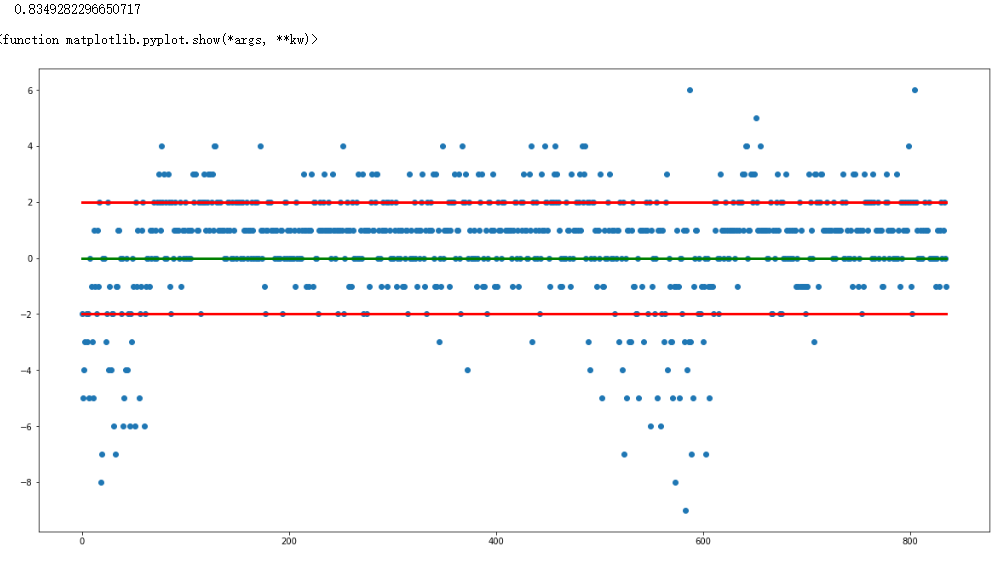可以看出在允许的误差范围2以内，其预测准确率达到83.5%，表明训练的模型是可用的。上图是是测试结果图，图中蓝点纵坐标表示预测值与真实值之差，横坐标为样本编号，绿色横线为Y=0的水平线，在该线上的点表示预测完全准确，遗憾的是允许误差为0的准确率仅为20.93%，上下红色横线之间代表在允许误差内预测正确的点，从该图中可以看出在数据集前端和中部有部分数据测试异常偏离，这或许可以成为该模型的改进点。
本次实战到此暂告一段落，初次撰写博文，也是初次涉及机器学习实战，必定有诸多不足甚至错误之处，还望看官指正。

展开全文线性回归
• 【机器学习】吴恩达机器学习视频作业-逻辑回归二分类 1 【机器学习】吴恩达机器学习视频作业-逻辑回归二分类 II 视频讲解如下： 彻底搞定机器学习算法理论与实战——逻辑回归-Logistic Regression 视频原地址...
使用逻辑回归进行二分类案例： 【机器学习】吴恩达机器学习视频作业-逻辑回归二分类 1 【机器学习】吴恩达机器学习视频作业-逻辑回归二分类 II
使用逻辑回归进行多分类
对于此练习，将使用逻辑回归算法手写数字（0到9）识别。 扩展上个练习中逻辑回归的实现，并将其应用于一对多的分类。源数据是在MATLAB的数据格式，需要使用一个SciPy读取。
主要思路：one vs all ，假如需要去将数据样本分为5类，就会创建5个逻辑回归模型。每个逻辑回归模型中，一种分类设置标签为1，即为正样本，其它类别标签设置为0，即为负样本，依此类推构建5个分类模型。预测时，将一条数据在5个模型中运行，得到在各个模型中分类的概率结果，再判断哪个数值最大，那么就认为是哪一类。
1 加载数据与数据可视化
import numpy as np
import pandas as pd
import matplotlib.pyplot as plt
from scipy.io import loadmat   # 加载matlab数据
%matplotlib inline

加载数据，数据内容如下。
data = loadmat('ex3data1.mat')   # 加载数据
data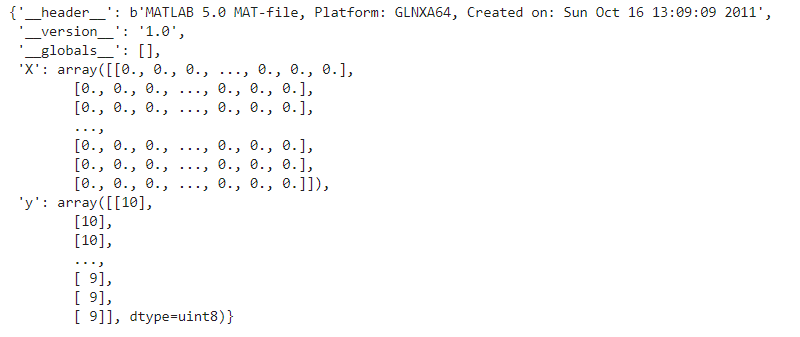查看样本数据，一共5000个样本，每个样本为400列，也就是说将一张图片（20*20）被压平了。
data['X'].shape, data['y'].shape
"""
((5000, 400), (5000, 1))
"""

查看分类标签有哪些值，其中数值0使用标签10代替。
np.unique(np.array(data['y']))   # 查看标签有哪些值
"""
array([ 1,  2,  3,  4,  5,  6,  7,  8,  9, 10], dtype=uint8)
"""

可视化数据，数据分布：0-499为数字0，标签为10，500-999为数字1，标签为1，以此类推。
plt.imshow(data['X'].reshape(20, 20))   # 查看数据图形
data['y']                               # 对应标签
"""
array(, dtype=uint8)
"""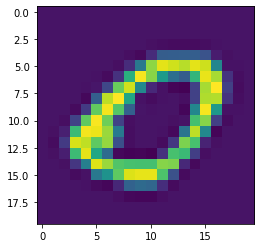plt.imshow(data['X'].reshape(20, 20))
data['y']
"""
array(, dtype=uint8)
"""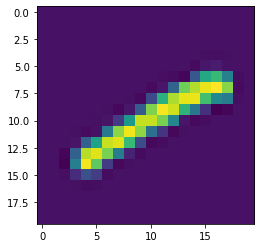plt.imshow(data['X'].reshape(20, 20))
data['y']
"""
array(, dtype=uint8)
"""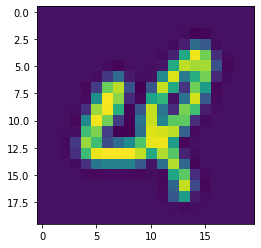2 构建sigmoid函数以及线性回归函数
模型函数创建，特征对应的线性函数如下： Expected node of symbol group type, but got node of type ordgroup 逻辑回归模型函数如下：

h

θ

(

x

)

=

g

(

θ

T

x

)

h_\theta(x) = g(\theta^T x)

其中g(z)表达式如下：

g

(

z

)

=

1

1

+

e

−

z

g(z) = \frac{1}{1+e^{-z}}

模型使用，我们预测：
当

h

θ

(

x

)

≥

0.5

h_\theta(x) \ge 0.5

时，预测

y

=

1

y=1

当

h

θ

(

x

)

<

0.5

h_\theta(x) < 0.5

时，预测

y

=

0

y=0

在多分类中，选择模型模型结果最大的那个类别。
# 定义一个sigmoid函数
def sigmoid(z):
# z可以是一个数，也可以是np中的矩阵
return 1.0/(1+np.exp(-z))
def model(X, theta):
# 单个数据样本维一个行向量 n个特征， theta为长度为n的行向量
# 当X为m行n列的数据，返回结果为m行1列的数据
theta = theta.reshape(1, -1)  # 转成符合矩阵计算
return sigmoid(X.dot(theta.T))

3 正则化损失函数(Regularize cost)

J

(

θ

)

=

1

m

∑

i

=

1

m

[

−

y

(

i

)

log

⁡

(

h

θ

(

x

(

i

)

)

)

−

(

1

−

y

(

i

)

)

log

⁡

(

1

−

h

θ

(

x

(

i

)

)

)

]

+

λ

2

m

∑

j

=

1

n

θ

j

2

J(\theta)=\frac{1}{m} \sum_{i=1}^{m}\left[-y^{(i)} \log \left(h_{\theta}\left(x^{(i)}\right)\right)-\left(1-y^{(i)}\right) \log \left(1-h_{\theta}\left(x^{(i)}\right)\right)\right]+\frac{\lambda}{2 m} \sum_{j=1}^{n} \theta_{j}^{2}

特点：比普通的损失函数多了一项

λ

2

m

∑

j

=

1

n

θ

j

2

\frac{\lambda}{2 m} \sum_{j=1}^{n} \theta_{j}^{2}

，注：此时j从1开始，在之前的损失函数基础上进行改进即可
# 损失函数
def cost(theta, X, y, l=1):
# X为特征数据集 单个特征数据为行向量形状要为mxn
# y为标签数据集 为一个列向量形状要为mx1
# theta为一个行向量 形状要为1xn
# l 正则化参数，这里默认设置为1
# 返回一个标量
left = -y*np.log(model(X, theta))
right = (1-y)*np.log(1 - model(X, theta))
return np.sum(left - right)/len(X) + l*np.power(theta[1:], 2).sum()/(2*X.shape)


查看单个模型训练数据状况。

# 初步查看数据
X = np.array(data['X'])
X = np.insert(X, 0, values=1, axis=1)    # 插入常数项
y = np.array(data['y']).reshape(-1, 1)
y_0 = np.array([1 if label == 0 else 0 for label in y]) # 数据样本中类为1设置为1，其它设置为0
class_num = 0                            # 获取分类个数
all_theta = np.zeros((10, X.shape))   # 生成10*(params + 1)维得参数，因为10个分类，每一个分类对应一组参数theta
X.shape, y.shape, all_theta.shape
"""
((5000, 401), (5000, 1), (10, 401))
"""

模型1初始损失函数
# 模型1的初始损失函数
cost(all_theta, X, y_0)
"""
3465.7359027995562
"""

4 梯度函数
正则化梯度公式分为两部分：

∂

J

(

θ

)

∂

θ

0

=

1

m

∑

i

=

1

m

(

h

θ

(

x

(

i

)

)

−

y

(

i

)

)

x

j

(

i

)

for

j

=

1

\frac{\partial J(\theta)}{\partial \theta_{0}}=\frac{1}{m} \sum_{i=1}^{m}\left(h_{\theta}\left(x^{(i)}\right)-y^{(i)}\right) x_{j}^{(i)} \quad \text { for } j = 1

∂

J

(

θ

)

∂

θ

j

=

(

1

m

∑

i

=

1

m

(

h

θ

(

x

(

i

)

)

−

y

(

i

)

)

x

j

(

i

)

)

+

λ

m

θ

j

for

j

≥

1

\frac{\partial J(\theta)}{\partial \theta_{j}}=\left(\frac{1}{m} \sum_{i=1}^{m}\left(h_{\theta}\left(x^{(i)}\right)-y^{(i)}\right) x_{j}^{(i)}\right)+\frac{\lambda}{m} \theta_{j} \quad \text { for } j \geq 1

# 参数的梯度
# X为特征数据集 mxn
# y为标签数据集 mx1
# l 正则化参数，默认为1
# theta为一个行向量 1xn
grad = ((model(X, theta) - y).T@X).flatten()/ len(X)
# 返回行向量


查看模型1初始梯度值
gradient(all_theta, X, y_0[:, np.newaxis])[:5]
"""
array([ 4.00000000e-01,  0.00000000e+00,  0.00000000e+00, -7.74530186e-08,
4.91729643e-07])
"""

7 使用优化函数计算参数
使用scipy的optimize模块。
import scipy.optimize as opt
def one_vs_all(X, y, kinds, l):
# X为特征数据集 mxn(尚未插入常数项1)
# y为标签数据集 mx1
# l 正则化参数，默认为1
# theta为一个行向量 1xn
features = X.shape  # 获取特征个数
all_theta = np.zeros((kinds, features))  # 初始化10个模型的参数
for i in range(1, kinds + 1):
theta = np.zeros(features)  # 当前模型参数值
# one vs all 标签转换
y_i = np.array([ 1 if label == i else 0 for label in y])[:, np.newaxis] # 转成列向量
# 使用TNC 截断牛顿法最小化损失值
fmin = opt.minimize(fun=cost, x0=theta, args=(X, y_i, l), method='TNC', jac=gradient)
all_theta[i-1,:] = fmin.x  # 获取计算的参数值
return all_theta


开始训练，获取各模型的参数。
all_theta = one_vs_all(X, y, 10, 1)
all_theta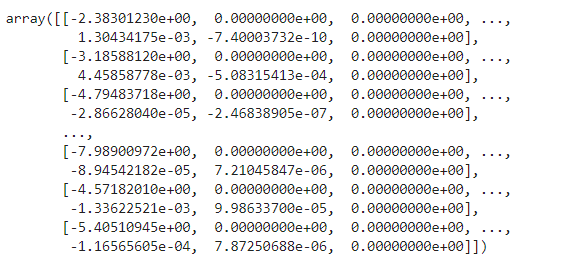8 使用训练的分类器预测数据
创建获取预测值函数。
def predict_all(X, all_theta):
# X 测试数据
# all_theta 模型参数
# 各模型计算结果后为一个5000x10的矩阵，从每行中选取最大值对应的索引即可
# 由于默认索引从0开始以及实际的标签是从1开始，需要给对应索引值+1
pre = np.argmax(sigmoid(X@all_theta.T), axis=1) + 1
return pre

查看模型预测的准确率。
y_pre = predict_all(X, all_theta)
# 比较各数据预测情况
correct = [1 if a==b else 0 for (a,b) in zip(y, y_pre)]
# 计算精度
accuracy = (sum(map(int, correct))/len(X))*100
print("accuracy:{}%".format(accuracy))
"""
accuracy:94.46%
"""

总结
相比于使用逻辑回归进行二分类来说，进行多分类也不是难题。但是如果类别多了，这种分类方式并不是一个很好的解决方法。程序源码可在订阅号"AIAS编程有道"中回复“机器学习”即可获取。
展开全文• 线性回归实战总结： 单变量线性回归： 1、加载和查看数据（准备工作） data = load(‘ex1data1.txt’); x=data(:,1); y=data(:,2); plot(x,y,’rx’,’markersize’,10); xlabel(‘population’); ...
线性回归实战总结：  单变量线性回归：  1、加载和查看数据（准备工作）

data = load(‘ex1data1.txt’);  x=data(:,1);  y=data(:,2);  plot(x,y,’rx’,’markersize’,10);  xlabel(‘population’);  ylabel(‘profit’);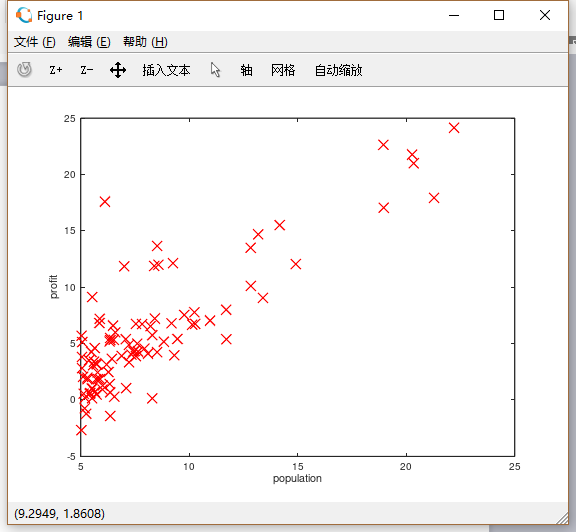2、定义代价函数（准备工作，递归下降的时候要调用）  定义输入：X，y，参数theta  单变量线性回归定义式：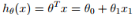这里可以看出参数为2*1矩阵，为方便矩阵计算，因为x的列数要等于θ的行数  所以假设x0=1，即θ0=θ0*x0，把所有x最左边加一列1，扩展为两列的X矩阵  m=length(x) //获得x矩阵的行数  X=[ones(m,1),x]; //构造一个跟x相同行数的全1的列向量，并和x合并成X矩阵  theta=zeros(2,1);//构造2*1的零矩阵theta  定义输出：计算在当前参数theta的情况下，系统的代价函数值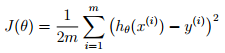function J = computeCost(X, y, theta)  J=0; //初始化代价函数值J  m=length(y) //计算样本个数，就是上式中的m，后边要用  predict = X*theta; //计算根据当前参数计算出的预测值  J = sum((predict-y).^2)/(2*m) //计算上式，返回代价函数值  end  3、递归下降法寻找参数局部最优解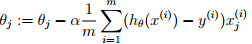（参数迭代方程）  定义迭代下降函数：  定义输入：X，y，theta(计算代价函数），alpha，iterations  其中α确定每次迭代的步长，这里暂设为0.01  还要指定迭代次数，因为可能永远迭代不完，暂定为1500次  alpha=0.01;  iterations=1500;  定义输出：theta（最终求得参数构造预测函数）、J_history（记录代价函数值，用于检验）  function [theta, J_history] = gradientDescent(X, y, theta, alpha, num_iters)  m = length(y);  J_history = zeros(num_iters,1);  for i = 1:num_iters  theta = theta-alpha/m*(X’*(X*theta-y));  J_history(i,1)=computeCost(X,y,theta);  end  end  在函数中调用：  [theta,J_history]=gradientDescent(X,y,theta,alpha,iterations);  4、检验迭代过程是否正确  绘制每一步迭代的代价函数值的变化图，看代价函数值是不是一直在下降：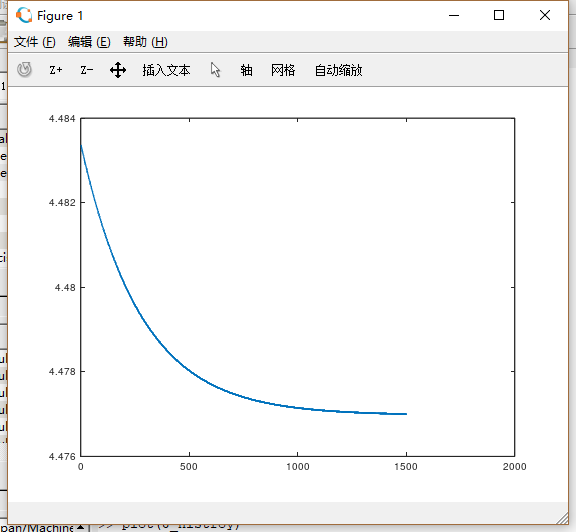将参数带入表达式，用表达式进行预测：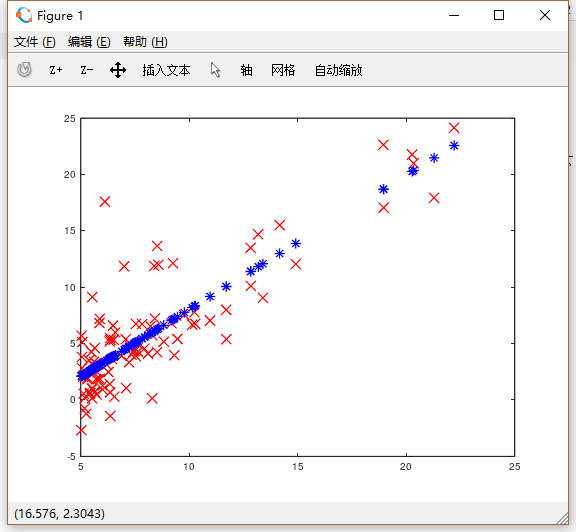按照预测方程将原始数据的属性带进去，得到预测值，发现就在那条直线上，然后与真实数据差异较大，因为真实数据可能本来也不是线性关系


展开全文笔记
• 吴恩达机器学习exp 1 (python) Programming Exercise 1: Linear Regression Machine Learning Linear regression with one variable 1.题目描述    In this part of this exercise, you will ...
• 视频讲解如下： 彻底搞定机器学习算法理论与实战——逻辑回归-Logistic Regression 视频地址：...二分类类型2 本文件的程序是基于吴恩达机器学习视频逻辑回归的作业，使用正则化的逻辑...
• 视频讲解如下： 彻底搞定机器学习算法理论与实战——逻辑回归-Logistic Regression 视频地址：...二分类类型1 本文件的程序是基于吴恩达机器学习视频逻辑回归的作业，使用的数据是ex...
• 文章目录0. 前言1. 集成方法2. 结合策略2.1. 平均法2.2.... 学习法3. 多样性度量4.... 如果这篇文章对你有一点小小的...同质集成中的个体学习器称为基学习器，相应学习算法称为基学习算法。 个体学习器的准确性越高，多样...集成学习 boosting bagging 监督学习
• 吴恩达机器学习第五个星期1.代价函数与反向传播1.1代价函数参考文献 本文是在学习吴恩达老师机器学习课程的基础上结合老师的ppt文献然后加上自身理解编写出来的，有些地方可能有遗漏或错误，欢迎大家批评指正。希望...神经网络监督学习 集成学习 adaboost boosting
• 这里小编简单的罗列出一些本人刚开始着手于机器学习时候的小问题，并将小编了解到的答案采用问答的方式给读者进行解释，本文也可以用做感兴趣的读者作为科普使用，如果答案有误，欢迎评论。先声明，小编也只是一个刚...
• python实现吴恩达机器学习线性回归ex.1 前述： 本节我们简单介绍下线性回归知识点，然后用常规方式完成作业，进一步引入矩阵将代码简单化。 首先，让咱们下载数据集： 数据集下载 提取码：0r1r 单线性回归 数据集...python 神经网络
•   本篇主要讲的是多变量的线性回归，从表达式的构建到矩阵的表示方法，再到损失函数和梯度下降求解方法，再到特征的缩放标准化，梯度下降...更多内容参考 机器学习&amp;深度学习 在平时遇到的一些问题，更多...多变量线性回归
• 第一章（可结合《机器学习实战》：https://blog.csdn.net/haoranhaoshi/article/details/81876539）： 优势在于机器的耐心和精力。 大量训练，使任务T由经验E得到性能度量P。 适合相当数量的或强或弱的相关属性，...
• 吴恩达机器学习第一个星期1.欢迎和介绍1.1机器学习的定义和类型1.2监督学习1.3无监督学习2.模型和代价函数2.1模型的表示2.2代价函数 第一个星期 1.欢迎和介绍 1.1机器学习的定义和类型 一个计算机程序被称为从经验E...
• 本篇介绍机器学习中的多分类和类别不平衡问题。 1. 多分类学习 一些算法可以直接进行多分类，而另一些算法则不行，基本思路是：将多分类任务拆为若干个二分类任务求解。 一对一（One vs. One, OvO）：给定数据集，...多分类 类别不平衡
• 文章目录0. 前言1. 划分选择1.1. ID3决策树1.2. C4.5决策树1.3. CART决策树2. 剪枝3. 连续值处理4.... 如果这篇文章对你有一点小小的帮助，请给个关注，点个赞喔，我...构建决策树算法如下图所示（图源：机器学习）： ...决策树 ID3 C4.5
• Week5 机器学习的应用技巧1.机器学习的应用2.机器学习诊断法2.1 评估数学模型2.2 学习曲线(Learning Curves)3.实战技巧3.1垃圾邮件分类器3.2 推荐的技巧3.3 常见的判别模型指标3.4 数据越多越好吗？ 1.机器学习的...笔记
• 规则学习是从训练数据中学习出一组能用于对未见示例进行判别的规则。 ⊕←f1∧f2∧...∧fL \oplus \leftarrow f_1\wedge f_2\wedge ...\wedge f_L ⊕←f1​∧f2​∧...∧fL​ 规则学习具有更好的可解释性，能更直观...规则学习 序贯覆盖 自顶向下 自底向上
• 如下图所示（图源：机器学习）： 2.3. TAN TAN（Tree Augmented naive Bayes）通过以下方式构建： 计算任意两个属性之间的条件互信息 I ( x i , x j ∣ y ) = ∑ x i , x j ; c ∈ C P ( x i , x j ∣ c )...贝叶斯 bayes
• sigmoid图像表示如下（图源：机器学习）： 1. 逻辑回归参数求解方法 使用 极大对数似然 定义代价函数： J = − 1 m ∑ i = 1 m ( y ( i ) log ⁡ y ^ ( i ) + ( 1 − y ( i ) ) log ⁡ ( 1 − y ^ ( i ) ) )...监督学习 逻辑回归
• 文章目录0. 前言 如果这篇文章对你有一点小小的帮助，请给个关注，点个赞喔，我会非常开心的~ 0. 前言监督学习 线性回归 正则化
• 高偏差高方差的学习曲线3. 调试模型顺序 如果这篇文章对你有一点小小的帮助，请给个关注，点个赞喔，我会非常开心的~ 0. 前言 泛化误差可分解为偏差、方差、噪声之和。 偏差：度量了学习算法的期望预测与真实...过拟合 欠拟合
• Bagging：对数据集进行有放回采样，采mmm次构成一个新的数据集，基于这个数据集训练基学习器，如此重复采样并训练直到达到指定学习器数目，将这些学习器集成 Bagging主要关注降低方差，因此在易受样本扰动的学习器...集成学习 随机森林 bagging
• 文章目录0. 前言1. EM算法2. 变分推断 如果这篇文章对你有一点小小的帮助，请给个关注，点个赞喔，我会非常开心的~ 0. 前言 EM算法是常用的估计参数隐变量的方法，它是一种迭代式算法，EM算法原型： ...概率图 变分推断
• 文章目录0. 前言1. 拉格朗日乘子法2. SVM参数求解方法3. 软间隔4. 核方法5.... ...严格的说，对超平面设置上界和下界，如下图所示（图源：机器学习）： 并满足： {wTxi+b⩾+1,&amp;amp;nbsp;y...支持向量机 SVM
• PCA算法如下图所示（图源：机器学习），基于线性变换进行降维： Z = W T X Z=W^TX Z = W T X ： 奇异值分解SVD ：通常可以使用SVD代替特征值分解： s v d = U Σ V T svd=U\Sigma V^T s v d = U Σ V T ， Σ \...降维 PCA 主成分分析
• 文章目录0. 前言1. 线性判别分析求解方法 如果这篇文章对你有一点小小的帮助，请给个关注，点个赞喔，我会非常开心的~ ...如下图所示（图源：机器学习）： 1. 线性判别分析求解方法 定义Xi&amp;amp;amp;amp;nb...线性判别分析 LDA 线性 分类...